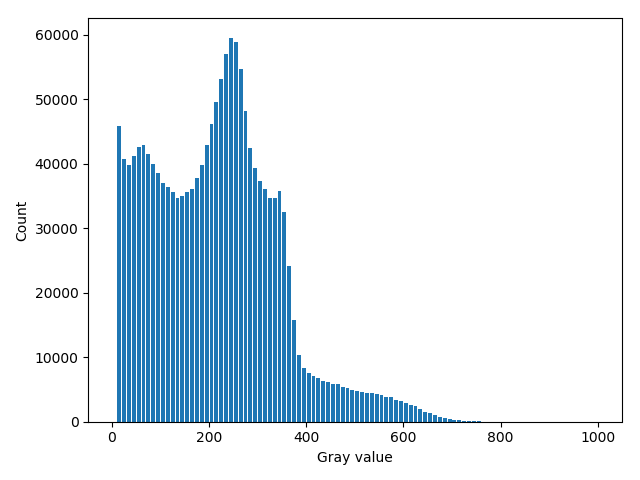# Histogram¶

This example shows how to calculate histogram of an image. In addition to the bin counts, the hist command outputs also bin starts.

In the end of the example the histogram is plotted using PyPlot.

def histogram():
"""
Demonstrates histogram calculation.
"""

# Calculate histogram
hist_min = 0        # Start of the first bin
hist_max = 1000     # End of the last bin
bin_count = 100     # Count of bins
hist = pi.newimage(ImageDataType.FLOAT32)
bins = pi.newimage(ImageDataType.FLOAT32)
pi.hist(img, hist, bins, hist_min, hist_max, bin_count)

# Get histogram data as NumPy array
data = hist.get_data()
bin_starts = bins.get_data()

# Most of the background pixels are in the first bin.
# We set it to zero so that the output image becomes nicely scaled
# automatically.
data = 0

# Bin edges can be generated like this if they have not been grabbed
# from hist(...) command
#bin_edges = np.linspace(hist_min, hist_max, bin_count + 1)

# Convert bin starts to bin centers
bin_size = bin_starts - bin_starts
bin_centers = bin_starts + bin_size / 2

# Plot the histogram
import matplotlib.pyplot as plt
plt.bar(bin_centers, data, 0.8*bin_size)
plt.xlabel('Gray value')
plt.ylabel('Count')
plt.tight_layout()
plt.show(block=False)
plt.savefig(output_file('histogram.png'))Histogram of the input image. The peak corresponding to the background has been erased.